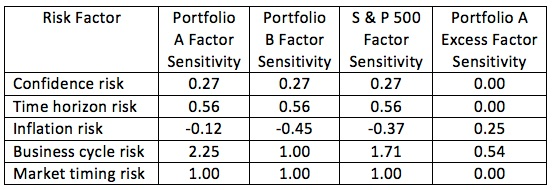### CFA Practice Question

There are 119 practice questions for this study session.

### CFA Practice Question

A portfolio manager uses the multifactor model shown in the following table:The S&P 500 is the benchmark for Portfolios A and B. The manager would like to use Portfolios A and B to construct a new portfolio that has zero excess business cycle factor sensitivity (relative to the business cycle sensitivity of the S&P 500). What is the inflation factor sensitivity of the resulting portfolio?
A. -0.838
B. -0.572
C. -0.263
Explanation: With w the weight on Portfolio A, (1 - w) the weight on Portfolio B, and 1.71 the sensitivity of the S&P 500 to the business cycle factor, we have

2.25w + 1.00 (1 - w) = 1.71 -> w = 0.568 (weight on Portfolio A), and 1 - w = 0.432 (weight on Portfolio B)

The resulting inflation factor sensitivity: 0.568 (-0.12) + 0.432 (-0.45) = -?0.263.